# Capacitor

### From DT Online

Capacitors are used to store electric charge which can be released into a circuit when required. The unit of capacitance is the farad which is a very large unit, named after Michael Faraday.

Most commonly used capacitors are rated in microfarads - µF (millionths).

Large value capacitors are often used as smoothing capacitors in A.C. power supplies. Other uses for capacitors are in timing circuits and filter circuits.

##### Capacitor Types

There are two main types of capacitor - polarised (or polarized) and non-polarised.

 Non-Polarised capacitors can be connected in a circuit either way round but they are normally only available in values up to 1µF. They can be constructed in a number of different materials and appear in different forms. Some of the more common ones are illustrated here.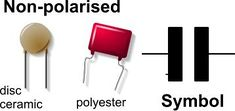Polarised capacitors must be connected with their "+" connection to the higher potential for them to function correctly. Elecrolytic capacitors are the most common polarised capacitors in use. The values of electrolytic capacitors starts at about 1µF and can extend to many thousands.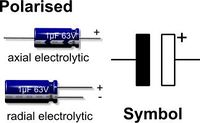Regard must be paid to the working voltage of a capacitor. This information is usually stamped on the device. A capacitor must not be subjected to a higher voltage than its working voltage.

Most capacitors have their value written upon their body but some polyester types use a colour code similar to the resistor colour code. Larger value electrolytic capacitors tend to suffer from relatively high leakage current. This means the charge on the capacitor gradually disappears through leakage, so using them in timing circuits can make the timing period inaccurate.

##### Capacitors in Circuits

Capacitors can be joined together in parallel.

In this arrangement the total capacitance is equal to the value of all the capacitors added together - i.e. : C(Total)= C1 + C2 + C3...etc

Capacitors may also be connected together end to end in series.

In this arrangement the total capacitance is calculated as follows:

___1___ = _______1______
C (Total)    C1 + C2 + C3...etc

or

C (Total) = _____C1xC2xC3____
C1xC2+C2xC3+C3xC1

##### Time Constant

Capacitors may also be connected together end to end in series with a resistor to create a resistor–capacitor circuit (RC circuit) in which a capacitor charges or discharges through a resistor for a known period of time known as the time constant

In this arrangement the time constant is calculated as follows: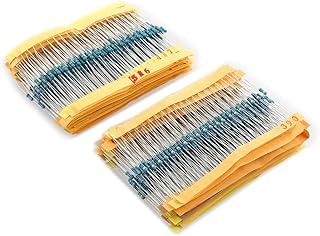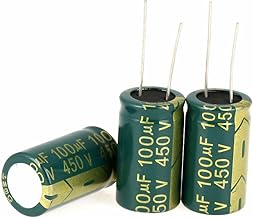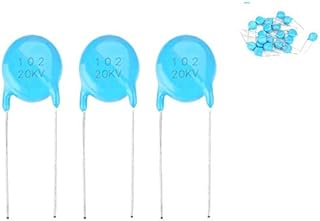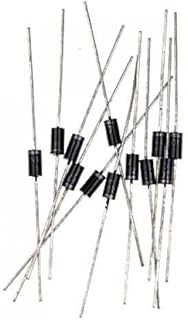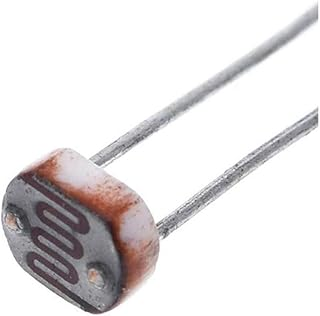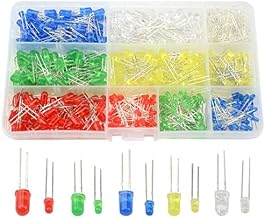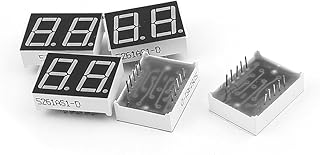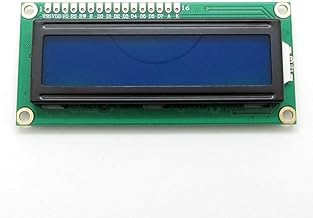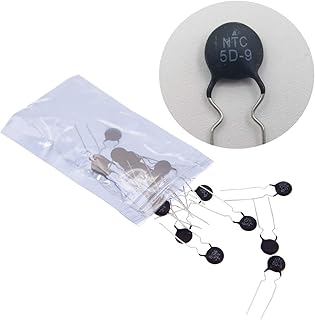1/4w Resistors PackElectrolytic CapacitorsCeramic Disc CapacitorsRectifier DiodesPhoto Light Sensitive Resistor (LDR)Light Emitting Diodes (LED)Common Cathode LED DisplayLCD Module DisplayThermistor Resistor